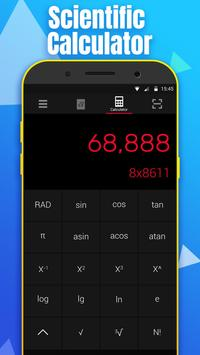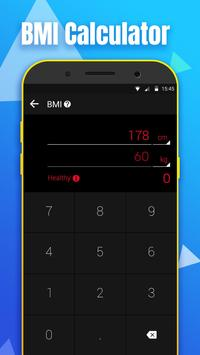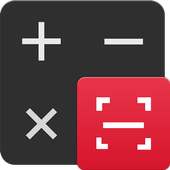##Math Calculator - Solve Math Problems by Camera   Version:  2.0.2

### Application Description:

Unlock Math Calculator to do maths solutions by taking photo! Math Calculator is a useful and easy-to-use calculator pro with basic calculator free and scientific calculator. This stylish calculator is also an equation solver and a great maths homework solver which can solve maths problems more efficiently.CAMERA .

This calculator app is developed by a experienced team which has already created a calculator app with 1,000,000+ install volume. We still devote to developing the best calculator app.
-Basic Calculator: Basic mathematics free functions with percentage.
-Maths Camera: Good maths homework helper which solves maths problems by taking photo.
-Solve Multiple Problems: Solve multi-line math problems at one time.
-Scientific Calculator: Based on casio 570.
-Calculation history : Never lose your calculations
-Equation calculator: Solve your maths homework efficiently.
-Great design: Nice user interface based on gesture.
-Floating Window: eep calculating above other apps.
-Vibrate and Sound: Fashion functions that simulates a real calculator.

## Conclusion

To conclude Math Calculator - Solve Math Problems by Camera works on Android operating system and can be easily downloaded using the below download link according to Freeware license. Math Calculator - Solve Math Problems by Camera download file is only 7.8 MB  in size.
Math Calculator - Solve Math Problems by Camera was filed under the Education category and was reviewed in softlookup.com and receive 5/5 Score.
Math Calculator - Solve Math Problems by Camera has been tested by our team against viruses, spyware, adware, trojan, backdoors and was found to be 100% clean. We will recheck Math Calculator - Solve Math Problems by Camera when updated to assure that it remains clean.

### Math Calculator - Solve Math Problems by Camera user Review

Please review Math Calculator - Solve Math Problems by Camera application and submit your comments below. We will collect all comments in an effort to determine whether the Math Calculator - Solve Math Problems by Camera software is reliable, perform as expected and deliver the promised features and functionalities.